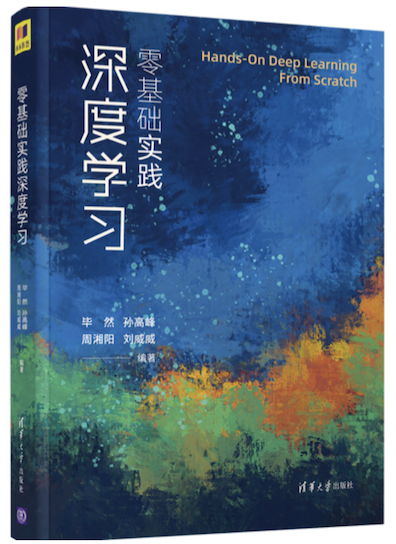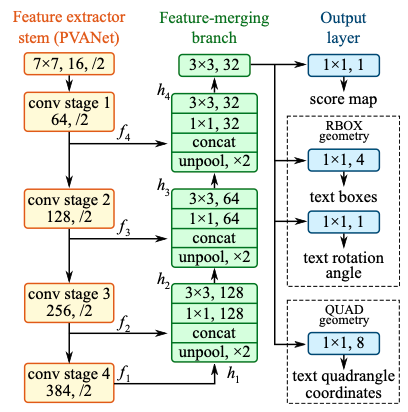# EAST¶

## 模型介绍¶

CTPN在水平文本的检测方面效果比较好，但是对于竖直方向的文本，或者多方向的文本，CTPN检测就很差。然而，在实际场景中，我们会遇到多种存在竖直方向文本的情况，例如很多书本封面的文本，如 图1 所示。>

## 模型结构¶

EAST的网络结构总共包含三个部分：Feature extractor stem（特征提取分支）, Feature-merging branch（特征合并分支） 以及 Output layer（输出层），网络结构如 图2 所示：1）特征提取分支

• 使用backbone网络提取特征，论文默认backbone为PVANet；

2）特征合并分支

• 上一步提取的feature map f1被最先送入unpool层(将原特征图放大２倍)；

• 然后与前一层的feature map f2进行拼接；

• 接着依次送入卷积核大小为1×1和3×3的卷积层，核数通道数随着层递减，依次为128，64，32；

• 重复上面三个步骤２次；

• 最后将经过一个卷积核大小为3×3，核数通道数为32个的卷积层；

3）输出层

• RBOX：主要用来预测旋转矩形的文本，包含文本得分和文本形状(AABB boundingbox 和rotate angle)，一共有６个输出，这里AABB分别表示相对于top，right，bottom，left的偏移；

• QUAD：用来预测不规则四边形的文本，包含文本得分和文本形状(８个相对于corner vertices的偏移)，一共有９个输出，其中QUAD有８个，分别为 $$$(x_{i},y_{i}),i\in[1,2,3,4]$$$。

## 模型loss¶

EAST损失函数由两部分组成，具体公式如下：

$L=L_{s}+λ_{g}L_{g}$

### 分数图损失¶

$L_{s} = -\beta Y^{*}log(\hat{Y})-(1-\beta)（1-Y^*）log(1-\hat{Y})$

#### 几何损失¶

• RBOX：IOU损失

$L_{AABB} = -log\frac{\hat{R}\cap R^*}{\hat{R}\cup R^*}$

$L_g=L_{AABB} + \lambda L_\theta$

$L_g = \min\limits_{\tilde{Q}\in P_{Q^*}} \sum\limits_{c_i \in C_Q, \tilde c_i \in C_\tilde Q}\frac{smoothed_{L_1}(c_i, \tilde{c}_i)}{8*N_{Q^*}}$

$N_{Q^*} = \min\limits_{i=1}^{4} D(p_i, p_{(i mode 4)+1})$

• 可以检测多方向的文本

• 不能检测弯曲文本